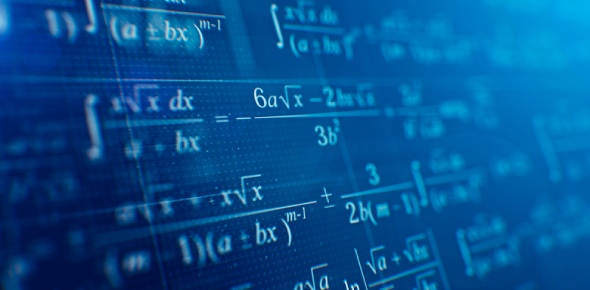# A Practice Quiz On General Mathematics

23 Questions | Total Attempts: 83SettingsHaving mathematics skills does not come easy and one has to ensure that they practice daily and master how to solve the different problems. The practice quiz below covers general mathematic problems and will help you perfect your skills. Give it a try and share your score in the comment section.

• 1.
Find the 22nd term of the Arithmetic Progression whose first term is 20 and common difference is 1/3.
• A.

27

• B.

27 1/3

• C.

27 2/3

• D.

28

• 2.
The graph of y = x2 lies entirely in the quadrants.
• A.

Q1 and Q3

• B.

Q1 and Q4

• C.

Q1 and Q2

• D.

Q2 and Q3

• 3.
If ( log a )/ 5 = ( log b )/6 =( log c )/7 , then b2
• A.

Ac

• B.

A*a

• C.

Bc

• D.

Ab

• 4.
If log10 (2x + 3) - 1= log10x , then find x.
• A.

2/7

• B.

3/4

• C.

7/8

• D.

3/8

• 5.
When a fair coin is tossed nine times, find the probability of getting heads at least once.
• A.

1/512

• B.

511/512

• C.

9/512

• D.

503/512

• 6.
In these series, you will be looking at both the letter pattern and the number pattern. Fill the blank in the middle of the series or end of the series. SCD, TEF, UGH, ____, WKL
• A.

CMN

• B.

UJI

• C.

VIJ

• D.

IJT

• 7.
Which symbol is needed to balance the scale?
• A.

Two Binoculars

• B.

Buoy and Anchor

• C.

Binoculars

• D.

Anchor

• 8.
Find the remainder when 6799 is divided by 7
• A.

4

• B.

6

• C.

1

• D.

2

• 9.
Two numbers are in the ratio 3:4. If their LCM is 240, the smaller of the two numbers is
• A.

100

• B.

80

• C.

60

• D.

50

• 10.
LCM of two numbers is 936. If their HCF is 4 and one of the number is 72, the other is
• A.

42

• B.

52

• C.

62

• D.

22

• 11.
A’s salary is 50% more than B’s. How much percent is B’s salary less than A’s?
• A.

33(1/4)%

• B.

33(1/3)%

• C.

33(1/2)%

• D.

33%

• 12.
Ramesh’s salary was reduced by 10% and then the reduced salary was increased by 10%. What was his ultimate loss?
• A.

5%

• B.

10%

• C.

1%

• D.

0%

• 13.
The price of milk was first increased by 10% and then decreased by 20%. What is the net percentage change in final price of milk?
• A.

12%

• B.

15%

• C.

10%

• D.

7.5%

• 14.
If a:b = 2:3 and b:c = 4:3, then find a:b:c
• A.

8:12:9

• B.

2:3:8

• C.

2:3:9

• D.

3:2:9

• 15.
Salaries of Ravi and Sumit are in the ratio 2:3. If the salary of each is increased by Rs 4000, the new ratio becomes 40:57. What is Sumit present salary.
• A.

32000

• B.

38000

• C.

36000

• D.

40000

• 16.
If 40% of a number is equal to two-third of another number, what is the ratio of first number to the second number.
• A.

2:5

• B.

2:7

• C.

5:7

• D.

5:3

• 17.
There are 30 people in a group. If all shake hands with one another , how many handshakes are possible?
• A.

870

• B.

435

• C.

30!

• D.

29! + 1

• 18.
If there are 15  Railway stations between Bangalore and Chennai, then how many general class tickets needs to be printed so as to enable a person to travel from anywhere to anywhere.
• A.

16! x 15!

• B.

15C2  x  2!

• C.

16 x 17

• D.

17C2 x 2!

• 19.
In how many ways can we arrange the word ‘FUZZTONE’ so that all the vowels come together?
• A.

1440

• B.

6

• C.

2160

• D.

4320

• 20.
A trekking group is to be formed having 6 members. They are to be selected from 3 girls, 4 boys and 5 teachers. In how many ways can the group be formed so that there are 3 teachers and 3 boys or 2 girls and 4 teachers?
• A.

55

• B.

90

• C.

27

• D.

144

• 21.
If  Surjan doesn’t want three vowels together, then in how many, can he arrange letters of the word 'MARKER'?
• A.

500

• B.

720

• C.

240

• D.

360

• 22.
In how many different ways can the letters of the word ‘GEOMETRY’ be arranged so that the vowels always come together?
• A.

720

• B.

4320

• C.

2160

• D.

40320

• 23.
If x:y = 1:3, then find the value of (7x+3y):(2x+y)
• A.

15:5

• B.

16:5

• C.

14:5

• D.

17:5

Related TopicsBack to top